# Completing the square

In algebra, completing the square is a way of rewriting a quadratic polynomial as the sum of a constant and a constant multiple of the square of a first-degree polynomial. Thus one has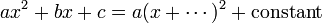$ax^2 + bx + c = a(x+\cdots)^2 + \text{constant}$

and completing the square is the way of filling in the blank between the brackets. Completing the square is used for solving quadratic equations (the proof of the well-known formula for the general solution consists of completing the square). The technique is also used to find the maximum or minimum value of a quadratic function, or in other words, the vertex of a parabola.

The technique relies on the elementary algebraic identity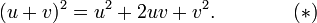$(u + v)^2 = u^2 + 2uv + v^2.\qquad\qquad(*)$

## Concrete examples

We want to fill in this blank:$3x^2 + 42x - 5 = 3(x+\cdots)^2 + \text{constant}.$

We write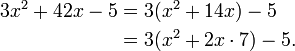\begin{align} 3x^2 + 42x - 5 & {} = 3(x^2 + 14x) - 5 \\ & {} = 3(x^2 + 2x\cdot 7) - 5. \end{align}

Now the expression ($x^2 + 2 x \cdot 7$) corresponds to$u^2 + 2 u v$ in the elementary identity labeled (*) above. If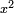$x^2$ is$u^2$ and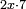$2 x \cdot 7$ is$2 u v$, then$v$ must be 7. Therefore$( u + v )^2$ must be$( x + 7 )^2$. So we continue:\begin{align} & {} 3(x^2 + 2x\cdot 7) - 5 \\ & {} = 3\left(x^2 + 2x\cdot 7 + 7^2\right) - 5 - 3(7^2), \end{align}

Now we have added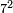$7^2$ inside the parentheses, and compensated (thus justifying the "=") by subtracting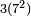$3 (7^2)$ outside the parentheses. The expression inside the parentheses is now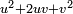$u^2 + 2 u v + v^2$, and by the elementary identity labeled (*) above, it is therefore equal to$( u + v )^2$, i.e. to$( x + 7 )^2$. So now we have$3(x + 7)^2 - 5 - 3(7^2) = 3(x + 7)^2 - 152.\,$

Thus we have the equality$3x^2 + 42x - 5 = 3(x + 7)^2 - 152.\,$

## More abstractly

It is possible to give a closed formula for the completion in terms of the coefficients a, b and c. Namely,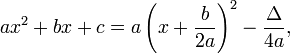$ax^2+bx + c = a\left(x + \frac{b}{2a}\right)^2 - \frac{\Delta}{4a},$

where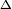$\Delta$ stands for the well-known discriminant of the polynomial, that is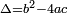$\Delta = b^2 - 4ac$.

Indeed, we have\begin{align} ax^2 + bx + c & {} = a\left( x^2 + \frac{b}{a}x \right) + c \\ & {} = a\left(x^2 + 2\frac{b}{2a}x\right) + c. \end{align}

The last expression inside parentheses above corresponds to$u^2 + 2 u v$ in the identity labelled (*) above. We need to add the third term,$v^2$: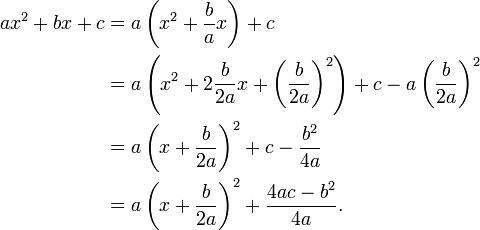\begin{align} ax^2 + bx + c & {} = a\left( x^2 + \frac{b}{a}x \right) + c \\ & {} = a\left(x^2 + 2\frac{b}{2a}x +\left(\frac{b}{2a}\right)^2 \right) + c - a\left(\frac{b}{2a}\right)^2 \\ & {} = a\left(x + \frac{b}{2a}\right)^2 + c - \frac{b^2}{4a} \\ & {} = a\left(x + \frac{b}{2a}\right)^2 + \frac{4ac - b^2}{4a}. \end{align}Some content on this page may previously have appeared on Citizendium.